Chapter 5 Class 12 Continuity and Differentiability

Class 12
Important Questions for exams Class 12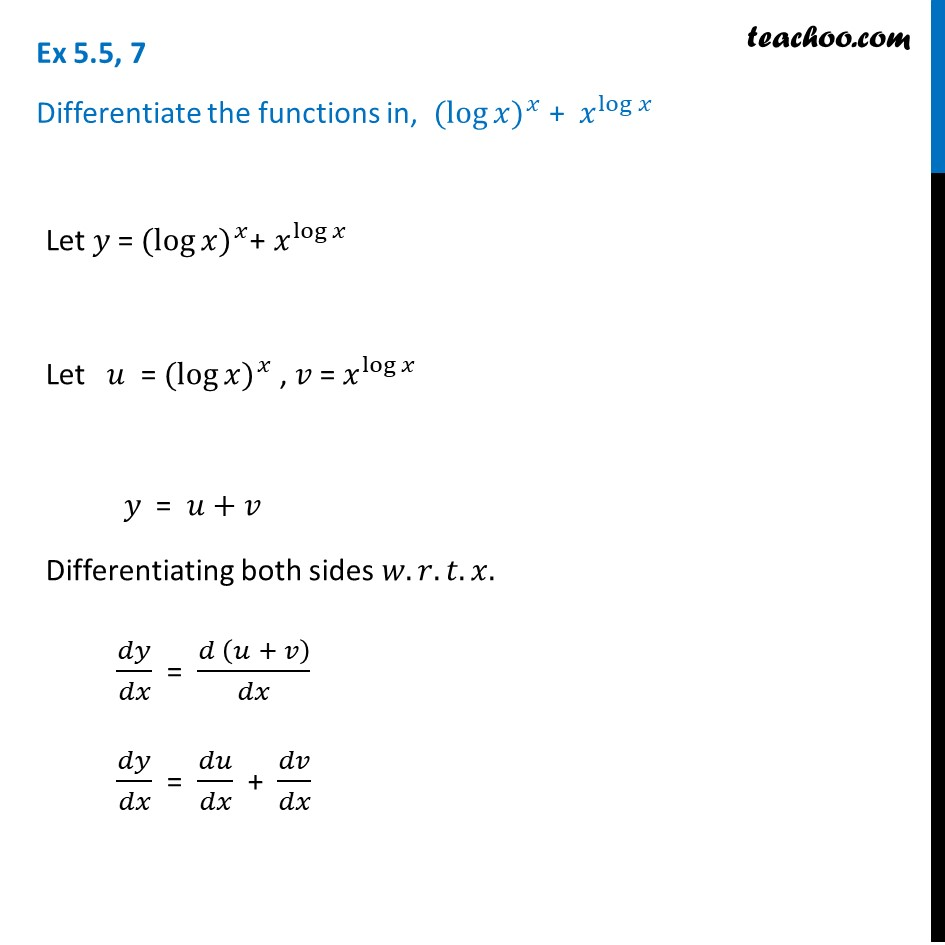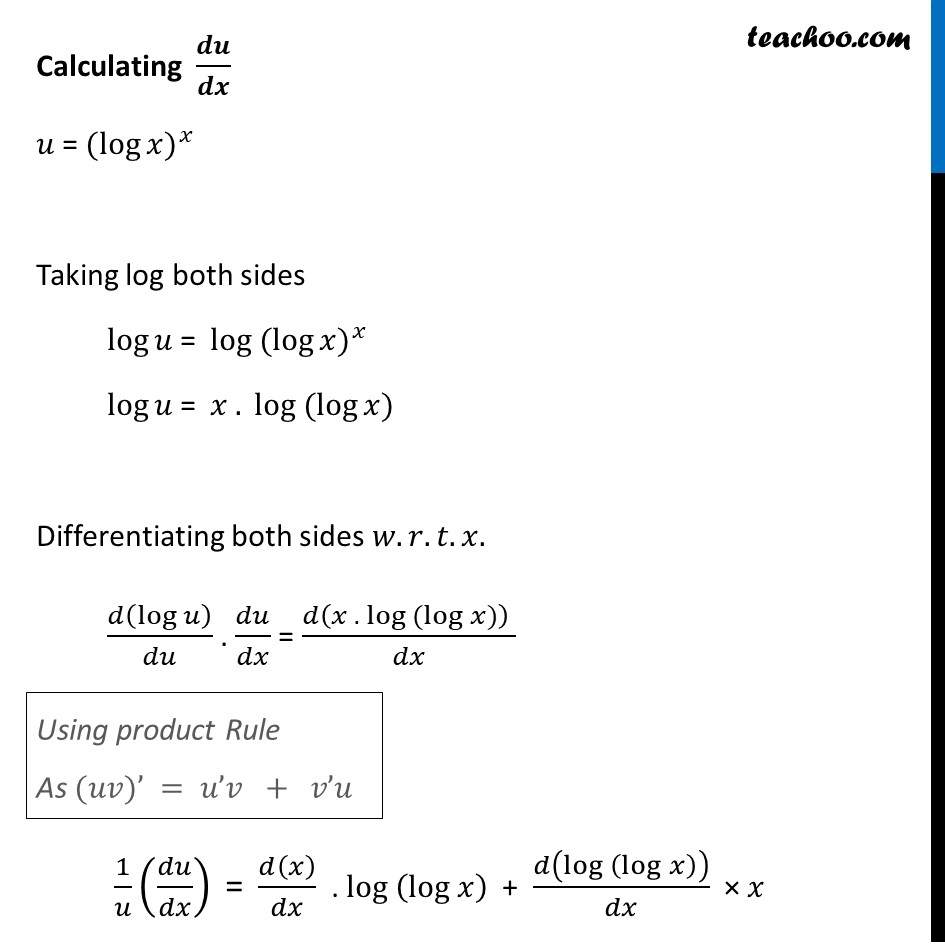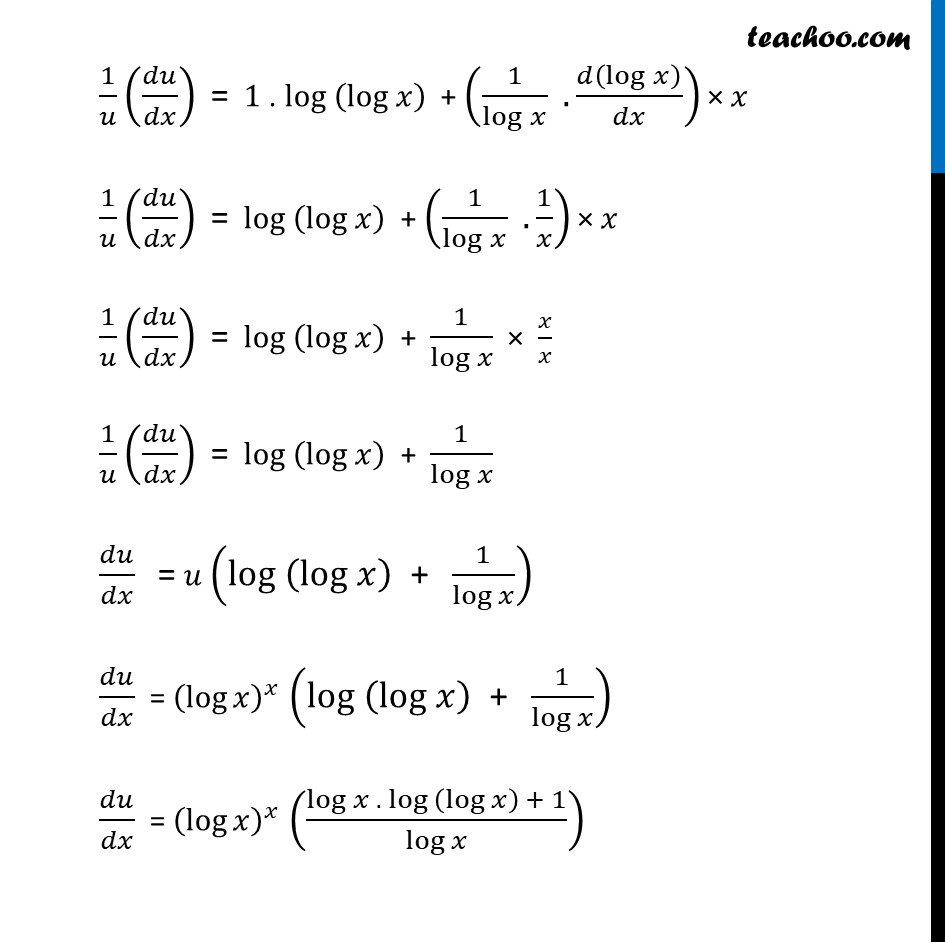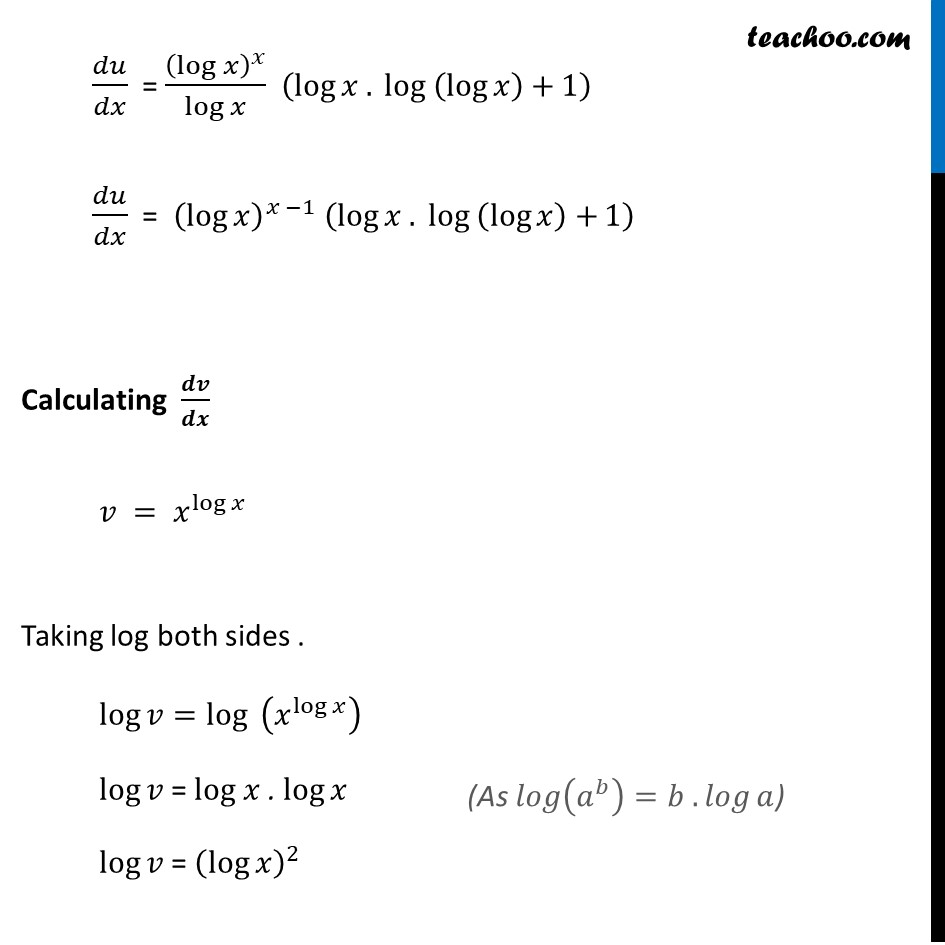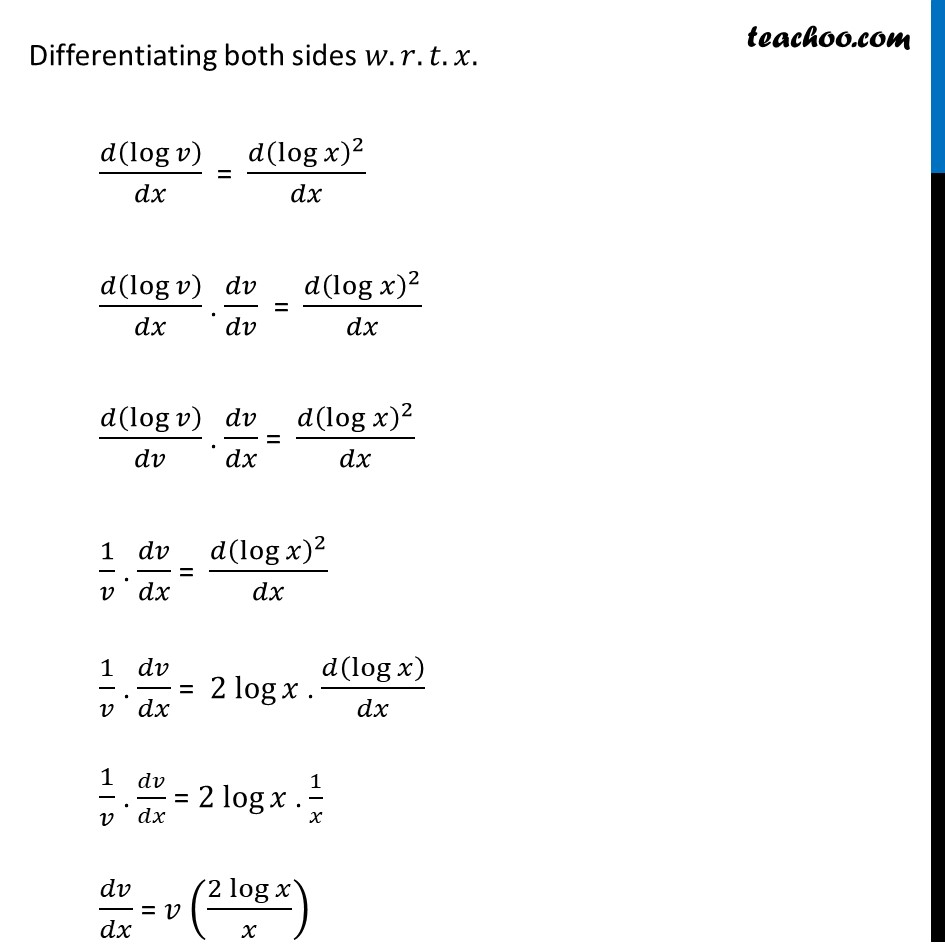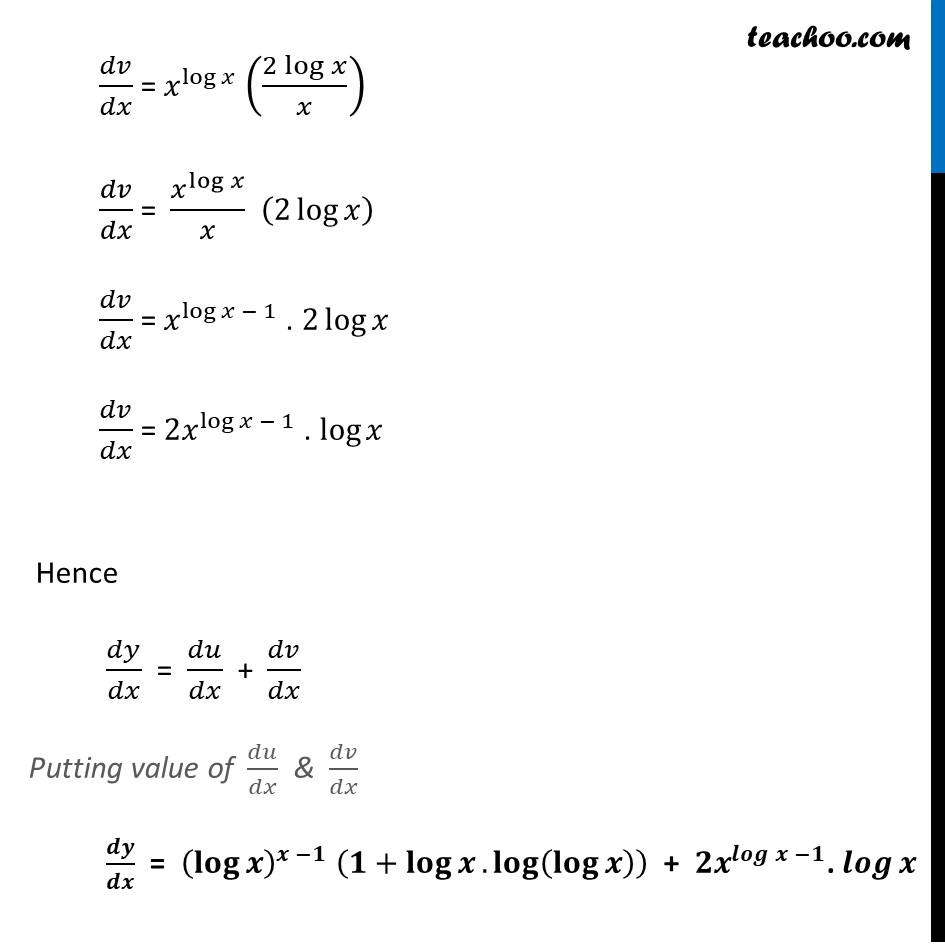Learn in your speed, with individual attention - Teachoo Maths 1-on-1 Class

### Transcript

Ex 5.5, 7 Differentiate the functions in, 〖(log⁡〖𝑥)〗〗^𝑥 + 𝑥^log⁡𝑥 Let 𝑦 = 〖(log⁡〖𝑥)〗〗^𝑥+ 𝑥^log⁡𝑥 Let 𝑢 = 〖(log⁡〖𝑥)〗〗^𝑥 , 𝑣 = 𝑥^log⁡𝑥 𝑦 = 𝑢+𝑣 Differentiating both sides 𝑤.𝑟.𝑡.𝑥. 𝑑𝑦/𝑑𝑥 = (𝑑 (𝑢 + 𝑣))/𝑑𝑥 𝑑𝑦/𝑑𝑥 = 𝑑𝑢/𝑑𝑥 + 𝑑𝑣/𝑑𝑥 Calculating 𝒅𝒖/𝒅𝒙 𝑢 = 〖(log⁡〖𝑥)〗〗^𝑥 Taking log both sides log⁡𝑢 = log 〖(log⁡〖𝑥)〗〗^𝑥 log⁡𝑢 = 𝑥 . log (log⁡〖𝑥)〗 Differentiating both sides 𝑤.𝑟.𝑡.𝑥. 𝑑(log⁡𝑢 )/𝑑𝑢 . 𝑑𝑢/𝑑𝑥 = (𝑑(𝑥 . log (log⁡〖𝑥)〗 ) )/𝑑𝑥 1/𝑢 (𝑑𝑢/𝑑𝑥)" =" 𝑑(𝑥)/𝑑𝑥 . log (log" " 𝑥) + 𝑑(log (log" " 𝑥))/𝑑𝑥 × 𝑥 Using product Rule As (𝑢𝑣)’ = 𝑢’𝑣 + 𝑣’𝑢 1/𝑢 (𝑑𝑢/𝑑𝑥)" =" 1 . log (log" " 𝑥) + (1/(log" " 𝑥) .𝑑(log" " 𝑥)/𝑑𝑥) × 𝑥 1/𝑢 (𝑑𝑢/𝑑𝑥)" =" log (log" " 𝑥) + (1/(log" " 𝑥) . 1/𝑥) × 𝑥 1/𝑢 (𝑑𝑢/𝑑𝑥)" =" log (log" " 𝑥) + 1/log⁡𝑥 × 𝑥/𝑥 1/𝑢 (𝑑𝑢/𝑑𝑥)" =" log (log" " 𝑥) + 1/log⁡𝑥 𝑑𝑢/𝑑𝑥 " =" 𝑢 (log (log" " 𝑥)" + " 1/log⁡𝑥 ) 𝑑𝑢/𝑑𝑥 = (log⁡𝑥 )^𝑥 (log (log" " 𝑥)" + " 1/log⁡𝑥 ) 𝑑𝑢/𝑑𝑥 = (log⁡𝑥 )^𝑥 ((log⁡〖𝑥 . 〖log 〗⁡(log⁡𝑥 ) +〗 1)/log⁡𝑥 ) 𝑑𝑢/𝑑𝑥 = (log⁡𝑥 )^𝑥/log⁡𝑥 (log⁡〖𝑥 . 〖log 〗⁡(log⁡𝑥 )+〗 1) 𝑑𝑢/𝑑𝑥 = (log⁡𝑥 )^(𝑥 −1) (log⁡〖𝑥 . 〖log 〗⁡(log⁡𝑥 )+〗 1) Calculating 𝒅𝒗/𝒅𝒙 𝑣 = 𝑥^log⁡𝑥 Taking log both sides . log⁡𝑣=log⁡〖 (𝑥^log⁡𝑥 )〗 log⁡𝑣 = log 𝑥 . log⁡𝑥 log⁡𝑣 = (log⁡𝑥 )^2 (As 𝑙𝑜𝑔⁡(𝑎^𝑏 )=𝑏 . 𝑙𝑜𝑔⁡𝑎) Differentiating both sides 𝑤.𝑟.𝑡.𝑥. 𝑑(log⁡𝑣 )/𝑑𝑥 = (𝑑(log⁡𝑥 )^2)/𝑑𝑥 𝑑(log⁡𝑣 )/𝑑𝑥 . 𝑑𝑣/𝑑𝑣 = (𝑑(log⁡𝑥 )^2)/𝑑𝑥 𝑑(log⁡𝑣 )/𝑑𝑣 . 𝑑𝑣/𝑑𝑥 = (𝑑(log⁡𝑥 )^2)/𝑑𝑥 1/𝑣 . 𝑑𝑣/𝑑𝑥 = (𝑑(log⁡𝑥 )^2)/𝑑𝑥 1/𝑣 . 𝑑𝑣/𝑑𝑥 = 2 log⁡𝑥 . 𝑑(log⁡𝑥 )/𝑑𝑥 1/𝑣 . 𝑑𝑣/𝑑𝑥 = 2 log⁡𝑥 . 1/𝑥 𝑑𝑣/𝑑𝑥 = 𝑣 ((2 log⁡𝑥)/𝑥) 𝑑𝑣/𝑑𝑥 = 𝑥^log⁡𝑥 ((2 log⁡𝑥)/𝑥) 𝑑𝑣/𝑑𝑥 = 𝑥^log⁡𝑥 /𝑥 (2 log⁡𝑥 ) 𝑑𝑣/𝑑𝑥 = 𝑥^(log⁡𝑥 − 1) . 2 log⁡𝑥 𝑑𝑣/𝑑𝑥 = 〖2𝑥〗^(log⁡𝑥 − 1) . log⁡𝑥 Hence 𝑑𝑦/𝑑𝑥 = 𝑑𝑢/𝑑𝑥 + 𝑑𝑣/𝑑𝑥 Putting value of 𝑑𝑢/𝑑𝑥 & 𝑑𝑣/𝑑𝑥 𝒅𝒚/𝒅𝒙 = (𝐥𝐨𝐠⁡𝒙 )^(𝒙 −𝟏) (𝟏+𝐥𝐨𝐠⁡𝒙.𝐥𝐨𝐠⁡(𝐥𝐨𝐠⁡𝒙 ) ) + 〖𝟐𝒙〗^(𝒍𝒐𝒈 𝒙 −𝟏). 𝒍𝒐𝒈⁡𝒙